## Tables index

#### Alhaji Bukar Abubakar, Mohammed B. Oumarou, Fasiu A. Oluwole, Sahabo Abubakar

Sustainable Energy. 2021, 9(1), 9-21 doi:10.12691/rse-9-1-2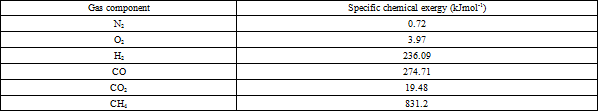• Table 1. Specific chemical exergy of gases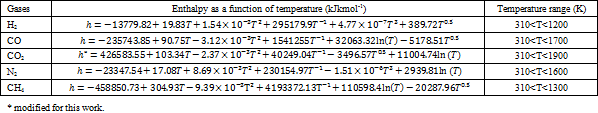• Table 2. Specific enthalpy of gases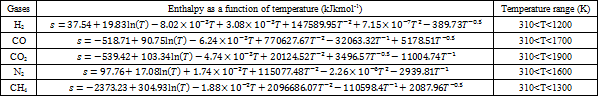• Table 3. Specific entropy of gases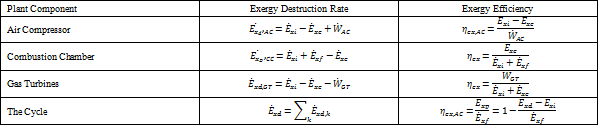• Table 4. Expression for Exergy Destruction rate and Exergy Efficiency for Different Components at Steady State Conditions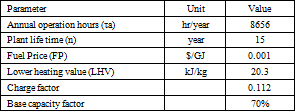• Table 5. Economic Data and Assumptions for the Study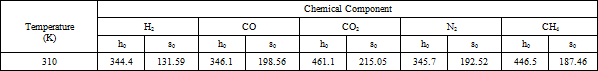• Table 6. Calculated Standard Specific Enthalpy and Standard Specific Entropy at 37°C (310K)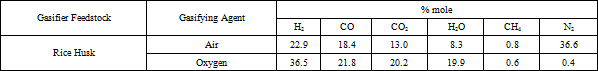• Table 7. Syngas Composition of Rice Husk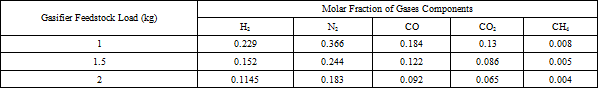• Table 8. Molar Fraction of Gas Components for Various Feedstock Loads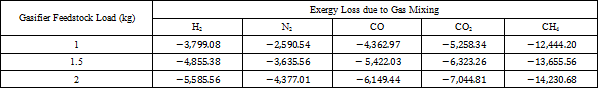• Table 9. Exergy Loss Due to Mixing at Various Feedstock Loads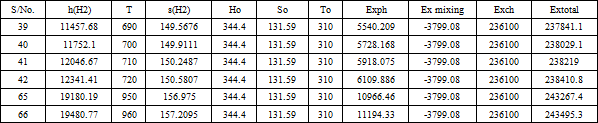• Table 10. Total Exergies for Hydrogen at 1kg load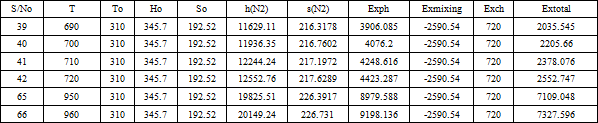• Table 11. Total Exergies for Nitrogen at 1kg load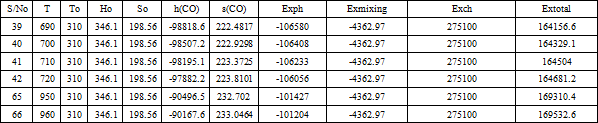• Table 12. Total Exergies for Carbon Monoxide at 1kg load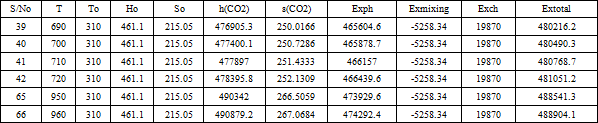• Table 13. Total Exergies for Carbon Dioxide at 1kg load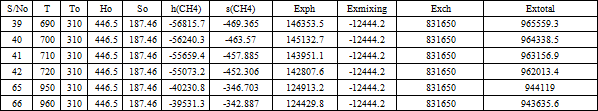• Table 14. Total Exergies for Methane at 1kg load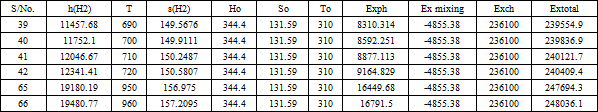• Table 15. Total Exergies for Hydrogen at 1.5 kg load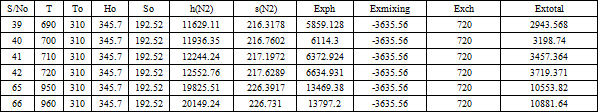• Table 16. Total Exergies for Nitrogen at 1.5 kg load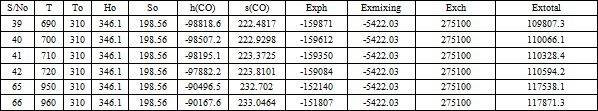• Table 17. Total Exergies for Carbon Monoxide at 1.5 kg load• Table 18. Total Exergies for Carbon Dioxide at 1.5 kg load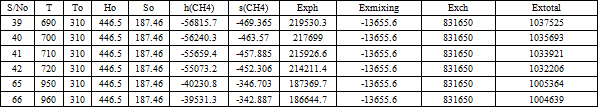• Table 19. Total Exergies for Methane at 1.5 kg load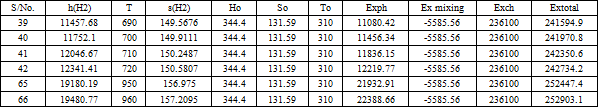• Table 20. Total Exergies for Hydrogen at 2 kg load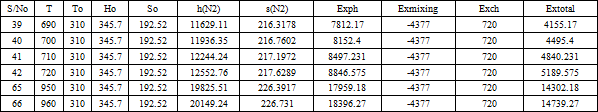• Table 21. Total Exergies for Nitrogen at 2 kg load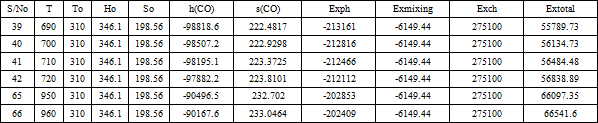• Table 22. Total Exergies for Carbon Monoxide at 2 kg load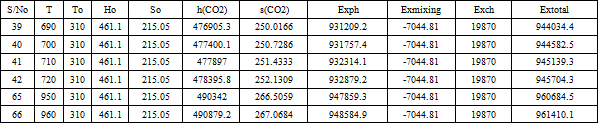• Table 23. Total Exergies for Carbon Dioxide at 2kg load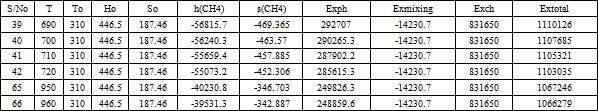• Table 24. Total Exergies for Methane at 2 kg load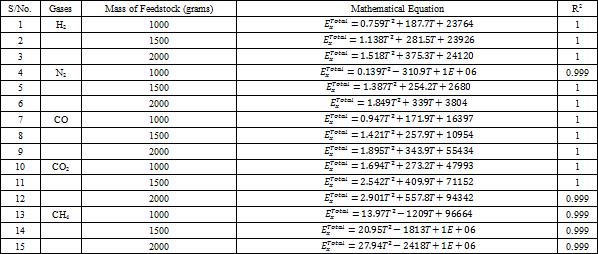• Table 25. Summary of Statistical Fits for the Exergy Analysis per Gas and Mass of Feedstock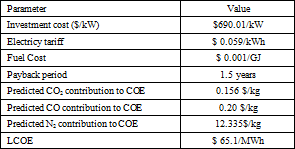• Table 26. Preliminary Exergoeconomic Analysis Results